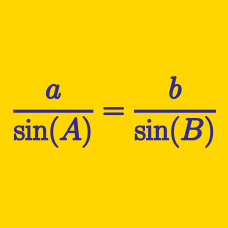Geometry

# Solving Triangles: Level 2 Challenges

If the angles of a triangle are in the ratio $4:1:1,$ then the ratio of the longest side to the perimeter is $\text{\_\_\_\_\_\_\_\_\_\_}.$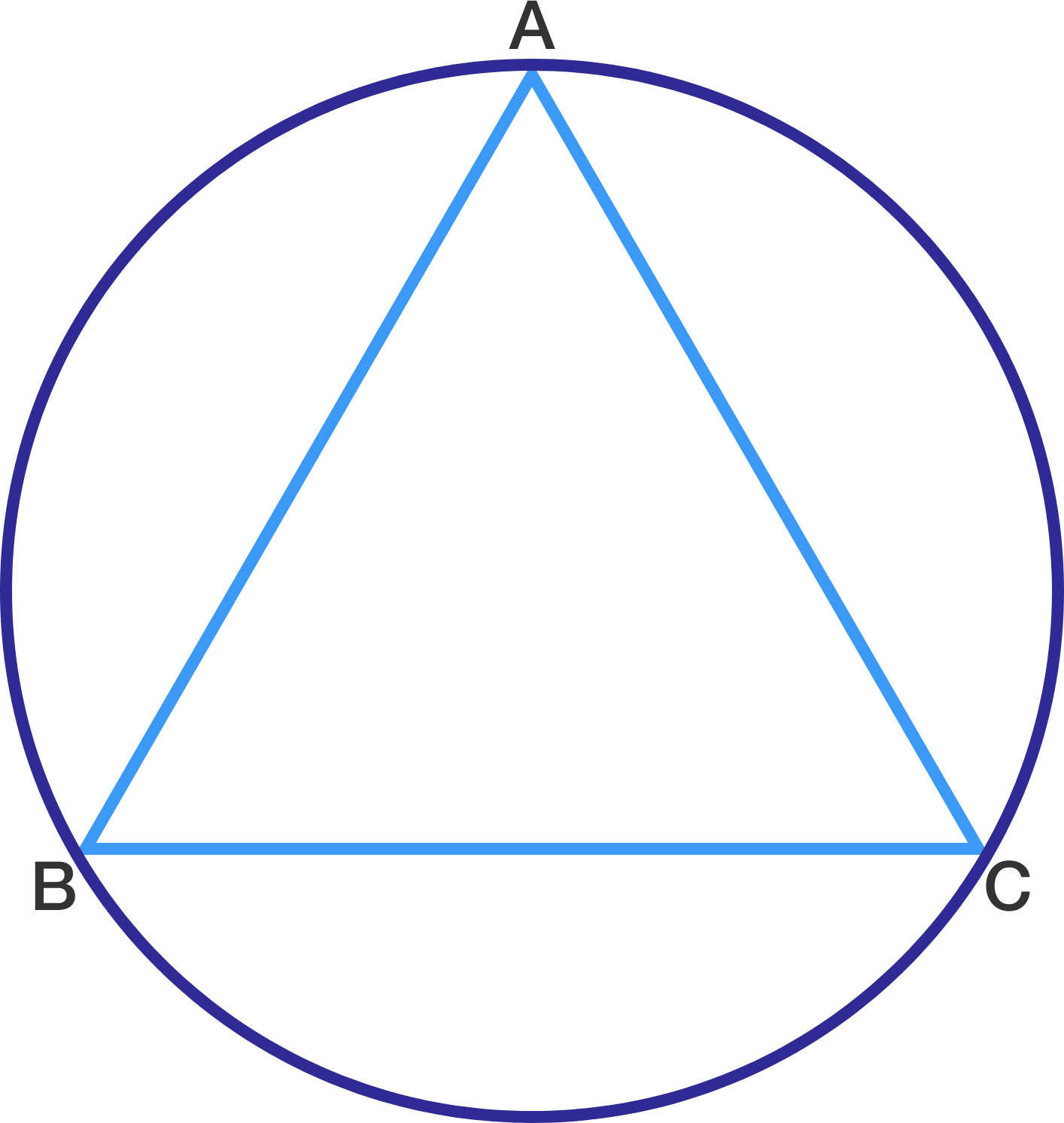In the figure, $\triangle ABC$ is an equilateral triangle where $AB = 6$ cm. The area of the circumcircle is $k\pi$ cm$^{2}$. Find the value of $k$.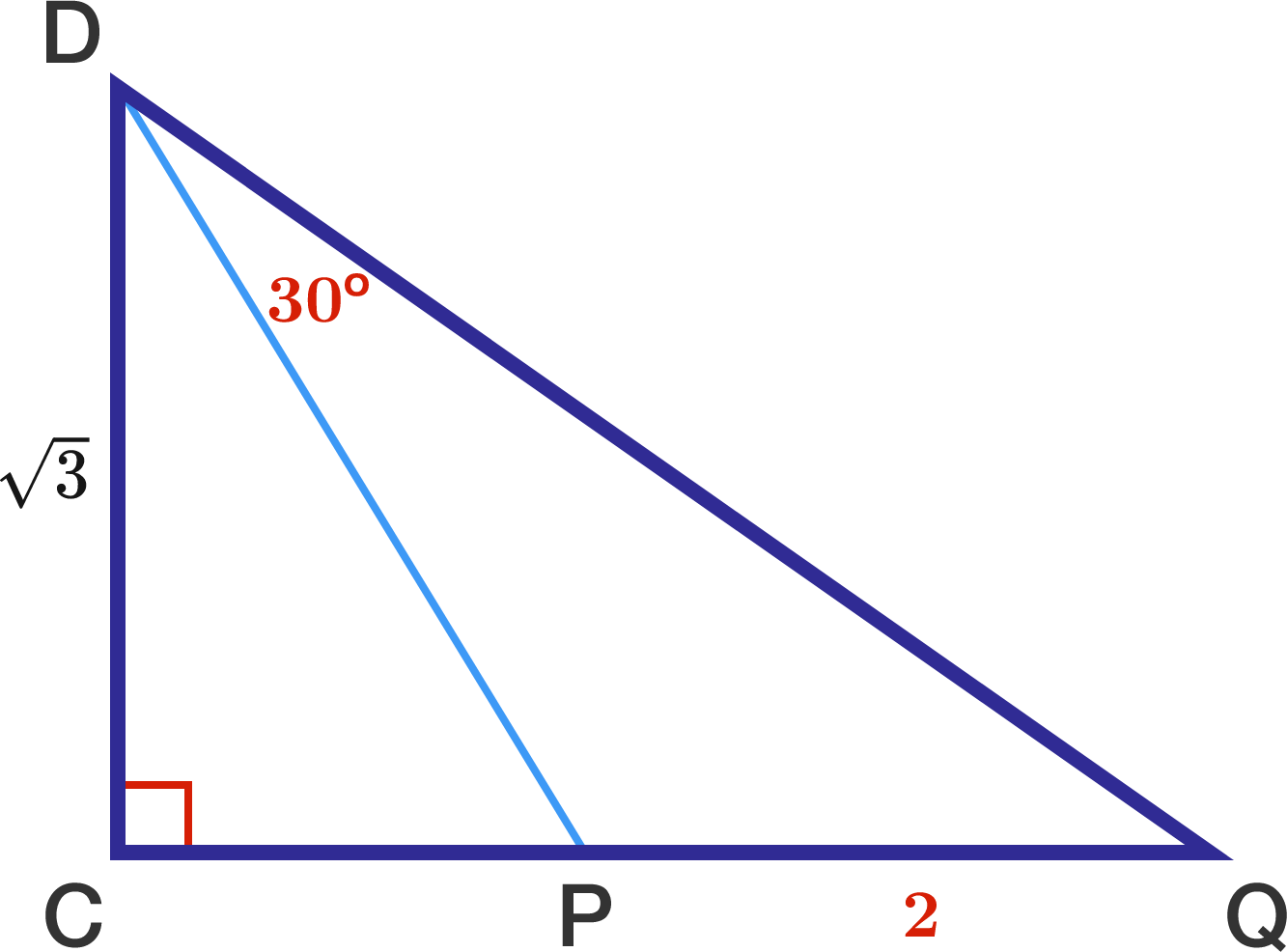If $CD=\sqrt{3},$ $PQ=2,$ and $\angle PDQ=30^\circ,$ find $CP.$

In triangle $ABC$, $\angle BAC = 2 \angle ABC$, $AB= 31, AC = 13$. What is $BC^2$?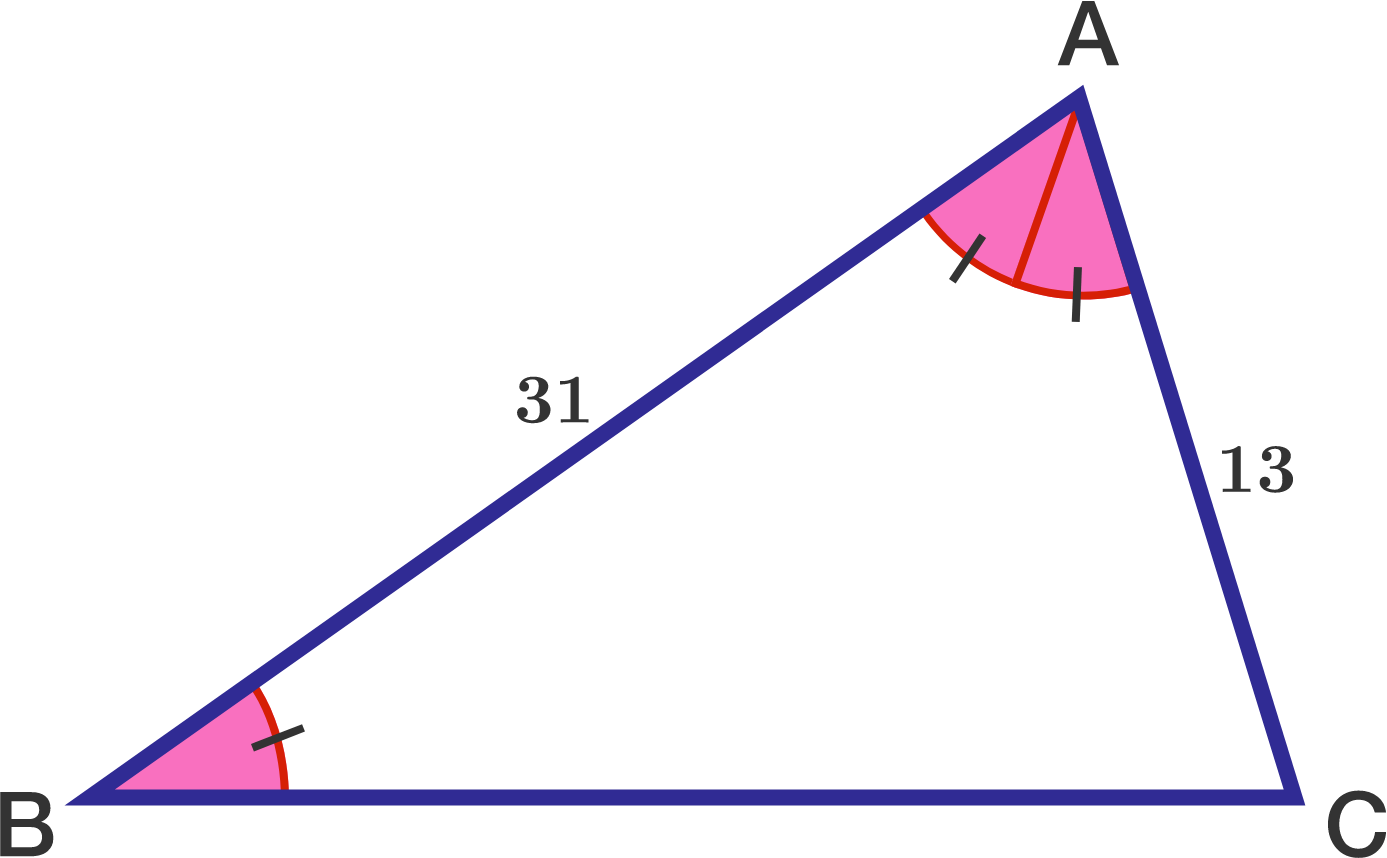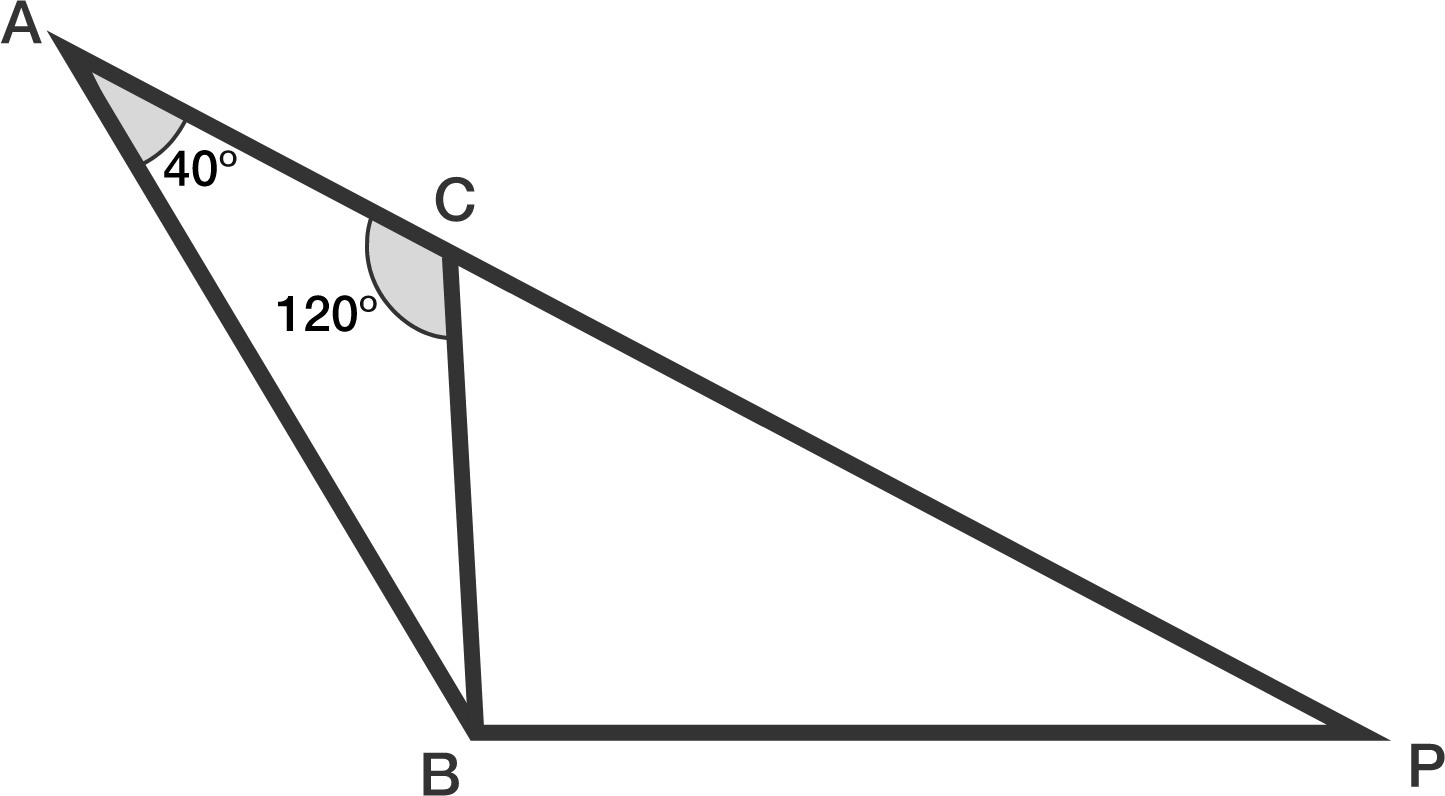If $AP = AC + 2\cdot CB$, what is the measure $\angle ABP$ in degrees?

×